# We Helped With This MATLAB Programming Homework: Have A Similar One?

SOLVEDCategory Programming MATLAB Undergraduate Solved Matlab Programming Experts

## Short Assignment Requirements

write the solutions in the command window and then copy it to word. It should be an easy homework. Please be as fast as possible. Thank you.

## Assignment Description

1.    (10 points) Consider the following matrices:

A =      3          2          8                                  B =      4          2          5          6

-5         6          0                                              -3        0          7          1

8          -4         9          16

a.    Determine the sum and product of each matrix column using built-in MATLAB matrix functions

b.    Multiply each element of A by 3 and divide each element of B by 4.

c.    Multiply matrix A by matrix B.  Save your answer as a new matrix, C

2.    (15 points) In heat transfer, radiation emitted from a black body (an object that does not reflect any light) is measured by the Stefan-Boltzmann law: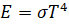Where E is the emittance (in W/m2), T is temperature (in Kelvin), and σ is the Stefan-Boltzmann constant, 5.67*10-8 W/(m2K4).  Write a function (.m file) that solves for the temperature of a black body that emits 6000 W/m2.  Round your answer to the nearest integer.

HINT: Use the nthroot function when solving for T.

3.    (20 points) Consider a generic chemical reaction, A à B + C, where a compound dissociates into two products.   The concentration, c, of component A is measured at 10-minute time (t) intervals, as shown in the table below:

 t, min 10 20 30 40 50 60 c, ppm 3.4 2.6 1.6 1.3 1 0.5

The engineer analyzing the data creates the following model equation to approximate the concentration for any value of t: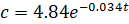It is common practice in science and engineering to plot discrete data points using symbols or markers, and to plot a mathematical model function using a continuous line. Therefore:

a.    Plot the data using magenta diamond-shaped markers

b.    Plot the model on the same graph using a solid green line

Be sure to include a legend, axis labels, axis limits, and an appropriate chart title.

4.    (25 points) The “butterfly curve” is a famous set of parametric equations, which are shown below: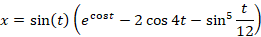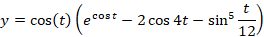a.    Create an array for t that ranges from 0 to 100 in intervals of 1/16 (or 0.0625)

b.    Generate x and y values using your t vector.  Note: You MUST use the element-by-element multiplication and exponentiation commands (.* & .^, respectively) rather than just the star (*) or carat (^)

c.    Plot x vs. t and y vs. t on the same figure.  Use a solid blue line x and a solid red line for y, along with a legend and appropriate chart & axis titles.

d.    Using the subplot command, stack your plot from part c on top of a plot of y vs. x.  Give this plot a title and axis labels as well. Can you see where the name “butterfly curve” comes from?!

5.    (25 points) The vapor pressure of a component as a function of temperature can be predicted from the Antoine Equation: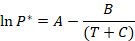Where:

A, B, and C are constants for a given component

T is the Temperature in Kelvin

P* is the Vapor Pressure in mm Hg

a.    Write a main program (.m file) in MATLAB that will calculate the vapor pressures of benzene between 300-600 Kelvin in increments of 30 K. The program should call a single function (separate .m file) called antoine, which will pass the Antoine constants and return (output) P* for each calculation of the vapor pressure.

The user should be prompted to enter a 1 or a 2 to select the type of loop used for the problem. Use a switch structure to solve the problem for either case - when case 1 is selected, a for loop should execute; when case 2 is selected, a while loop should run.  In both cases, store the values of the temperature and pressure in a matrix

b.    Use fprintf statements to print the your matrix as a table with the following headings:

Benzene

Temperature                Vapor Pressure

(deg. Celsius)                    (mm Hg)

Remember to convert from Kelvin to degrees Celsius.

c.    Finally, plot the vapor pressures as a function of temperature on a single plot, which is labeled appropriately.  Use data markers of your choosing rather than a continuous curve.

Constants for Antoine Equation:

A                                 B                     C

Benzene                                15.9008                      2788.1            -52.36

Is it free to get my assignment evaluated?

Yes. No hidden fees. You pay for the solution only, and all the explanations about how to run it are included in the price. It takes up to 24 hours to get a quote from an expert. In some cases, we can help you faster if an expert is available, but you should always order in advance to avoid the risks. You can place a new order here.

How much does it cost?

The cost depends on many factors: how far away the deadline is, how hard/big the task is, if it is code only or a report, etc. We try to give rough estimates here, but it is just for orientation (in USD):

 Regular homework \$20 - \$150 Advanced homework \$100 - \$300 Group project or a report \$200 - \$500 Mid-term or final project \$200 - \$800 Live exam help \$100 - \$300 Full thesis \$1000 - \$3000

How do I pay?

Credit card or PayPal. You don't need to create/have a Payal account in order to pay by a credit card. Paypal offers you "buyer's protection" in case of any issues.

Why do I need to pay in advance?

We have no way to request money after we send you the solution. PayPal works as a middleman, which protects you in case of any disputes, so you should feel safe paying using PayPal.

Do you do essays?

No, unless it is a data analysis essay or report. This is because essays are very personal and it is easy to see when they are written by another person. This is not the case with math and programming.

Why there are no discounts?

It is because we don't want to lie - in such services no discount can be set in advance because we set the price knowing that there is a discount. For example, if we wanted to ask for \$100, we could tell that the price is \$200 and because you are special, we can do a 50% discount. It is the way all scam websites operate. We set honest prices instead, so there is no need for fake discounts.

Do you do live tutoring?

No, it is simply not how we operate. How often do you meet a great programmer who is also a great speaker? Rarely. It is why we encourage our experts to write down explanations instead of having a live call. It is often enough to get you started - analyzing and running the solutions is a big part of learning.

What happens if I am not satisfied with the solution?

Another expert will review the task, and if your claim is reasonable - we refund the payment and often block the freelancer from our platform. Because we are so harsh with our experts - the ones working with us are very trustworthy to deliver high-quality assignment solutions on time.

Customer Feedback

"Thanks for explanations after the assignment was already completed... Emily is such a nice tutor! "

Order #13073